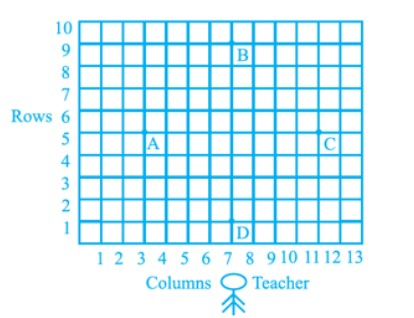#### Students of a school are standing in rows and columns in their playground for a drill practice. A, B, C and D are the positions of four students as shown in figure. Is it possible to place Jaspal in the drill in such a way that he is equidistant from each of the four students A, B, C and D? If so, what should be his position?Solution.         Points are A(3, 5), B(7, 9), C(11, 5), D(7, 1)

= 8

= 8
AB = BC = AD, AC = BD
Hence, ABCD is square
The diagonals cut each other at mid-point, which is the equidistance from all four corners of square.

(x1, y1) =(3, 5)             (x2, y2) = (11, 5)

This should be the position of Jaspal.